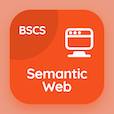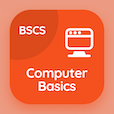Online CS Degree Courses

Digital Logic Design MCQs

Digital Logic Design MCQ - Topics

Learn Code Conversion Multiple Choice Questions (MCQ Quiz), Code Conversion quiz answers PDF to learn digital logic design course for digital logic design online classes. Combinational Logics Multiple Choice Questions and Answers (MCQs), Code Conversion quiz questions for top online computer science programs. Code Conversion Book PDF: subtractors in combinational logics, half adders, design procedure in combinational logics, multi level nor circuits test prep for master's degree in computer science.

"Code conversion circuits mostly uses" MCQ PDF: code conversion App APK with and-or gates, and gates, or gates, and xor gates choices for top online computer science programs. Study code conversion quiz questions for merit scholarship test and certificate programs for BSc computer science.

## MCQs on Code Conversion Quiz

MCQ: Code conversion circuits mostly uses

AND-OR gates
AND gates
OR gates
XOR gates

MCQ: The simplified expression of full subtractor borrow is

B=xy+xz+yz
B=xy'+xz'+yz
B=x'y+xy+xz
B=x'y+x'z+yz

MCQ: Sometimes it is necessary to use the output of one system as the

input to another
carry to another
borrow to another
subtraction

MCQ: If two systems have different codes then circuit inserted between them is

combinational circuit
sequential circuit
combinational sequence circuit
conversion circuit

### More Quizzes on Digital Logic Design Book